# Using a Peak-Current-Mode Controller to Design a Boost Converter

### 要約

This application note describes how to design boost converters using the MAX17597 and MAX17498B/C peak-current-mode controllers. Boost converters can be operated in discontinuous conduction mode (DCM) or continuous conduction mode (CCM). The operating mode can affect the component choices, stress level in power devices, and controller design. Formulas for calculating component values and ratings are presented.

### Boost Converter

Typical boost converter circuit schematics, built around the MAX17597 and MAX17498B/C peak-current-mode controllers, are shown in Figure 1 and Figure 2, respectively. Input capacitors C1 and C2, inductor LIN, MOSFET N1(internal for MAX17498B/C), diode D1, and output capacitor C8 form the main components for power conversion. C3 decides the soft-start duration. C4 decouples VDRV or VCC output voltage (set to 4.9V by the internal regulators). R1 programs the slope compensation, which is necessary to provide internal stability in peak-current-control scheme. R2 and R3 form the potential divider for output voltage feedback. The network R4, C5, C6 forms the closed-loop compensation network. The resistor network R5, R6, R7 sets the input enable and overvoltage threshold levels. R8 sets the switching frequency in the case of the MAX17597. The MAX17498B switches at 500kHz fixed switching frequency and MAX17498C switches at 250kHz. RCS senses the current in MOSFET N1 for the MAX17597 and Rcs = 0.5Ω for the MAX17498B/C, and filter components R9 and C7 provide leading-edge filtering of the sensed current signal, which is not required for the MAX17498B/C. RLIM is the current-limit resistor required for the MAX17498B/C. RGOOD and CRef components are required for PGOOD signal and reference voltage, respectively.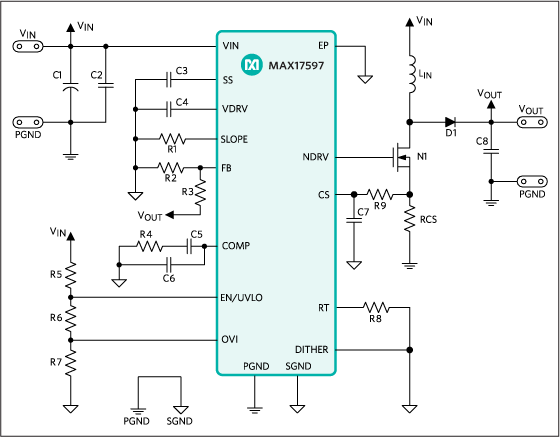Figure 1. Typical application circuit for MAX17597.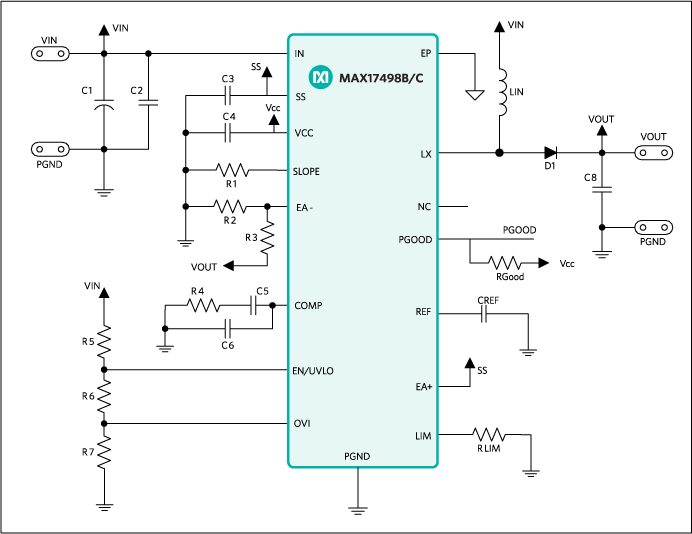Figure 2. Typical application circuit for MAX17498B/C.

### DCM Boost

In a DCM boost converter, the inductor current returns to zero in every switching cycle. Energy stored during the ON time of the main switch, MOSFET N1 in the case of the MAX17597, is entirely depleted within the switching cycle.

Inductor Selection

The design procedure starts with calculating the boost converter's input inductor (LIN), such that it operates in DCM at all operating input voltage and load current conditions. The critical inductance required to maintain DCM operation is calculated as:

 LIN ≤ [((VOUT - VINMIN) × VINMIN²) × η]/(2 × IOUT × VOUT² × fSW) henry (Eq. 1)

where VINMIN is the minimum input voltage, VOUT is the desired output voltage, IOUT is the load current specification, and fSW is the chosen switching frequency for MAX17597, fSW = 250kHz for MAX17498C and fSW = 500kHz for MAX17498B. η is expected efficiency in the range of 70% to 95% based on specifications. Practical LIN choice shall take into account tolerances and saturation effects.

Peak Current Limit

For the purpose of setting the current limit, the peak current in the inductor can be calculated as:in amperes (Eq. 2)

The value of current limit, in MOSFET N1, is set as:

 ILIM = IPK × 1.2 in amperes (Eq. 3)

In the case of the MAX17597, the current-sense resistor (RCS), connected between the source of MOSFET N1 and PGND, sets the peak current limit. The current-limit comparator has a voltage trip level (VCS-PEAK) of 300mV. Use the following equation to calculate the maximum value of RCS:

RCS = (300mV/ILIM

In the case of the MAX17498B/C the RLIM sets the current-limit value and its minimum value is:

RLIM = ILIM × 50 in kΩ (Note that the worst-case ILIM is 1.62A for the MAX17498B/C)

Output Capacitor Selection

The output capacitance (C8) can be calculated as follows:

 COUT = 1/2 × (ISTEP × TRESPONSE)/ΔVOUT in farads (Eq. 4)

Where TRESPONSE = (0.33/FC + 1/FSW) is the response time of the controller.

ISTEP is the load step expected at the output of boost converter, ΔVOUT is the allowable output voltage deviation for the expected load step, and fC is the target closed-loop crossover frequency. fC is chosen to be in the order of 1/10 of the switching frequency fSW. For the boost converter, the output capacitor supplies the load current when the main switch is ON, and therefore the output voltage ripple is a function of duty cycle and load current. Use the following equation to calculate the steady state output voltage ripple:

 ΔVOUT = (IOUT × LIN × IPK)/(VINMIN × COUT) in volts (Eq. 5)

Input Capacitor Selection

The minimum required input ceramic capacitor (C2) can be calculated based on the ripple allowed on the input DC bus.

 CIN = IPK/(ΔVIN × FSW× 8) (Eq. 6)

Where ΔVIN is the ripple voltage allowed on the input DC bus.

In practice, an electrolytic capacitor (C1) is provided to decouple any source inductance formed by the input cables. The electrolytic capacitor, C1, may also be used as an energy storage element, which can supply power when input power fails.

Capacitor values change with temperature and applied voltage. Refer to capacitor data sheets to select capacitors that would guarantee the required CIN and COUT values across the operating range. Use the worst-case derated value of capacitance, based on temperature range and applied voltage, for further calculations.

Error Amplifier Compensation Design

The loop compensation values for the error amplifier are calculated as (for R4, C5, and C6):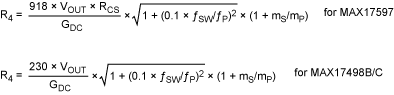(Eq. 7)

where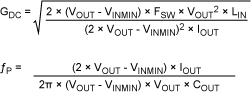(Eq. 8)

mS is the programmed slope (with default minimum slope = 50mV/µs for the MAX17597 and is 60mV/µs for the MAX17498B/C), and mP = VINMIN/L × RCS (Rcs = 0.5Ω for MAX17498B/C).

 C5 = 1/(2π × fP × R4) (Eq. 9)

 C6 = 1/(π × fSW × R4) (Eq. 10)

Slope Compensation

In theory, a DCM boost converter does not require slope compensation for stable operation. In practice the converter needs a minimum amount of slope for good noise immunity at very light loads. The minimum slope is set for the MAX17597 by allowing the SLOPE pin to float. The minimum slope compensation ramp is set to 50mV/µs for MAX17597 and 60mV/µs for MAX17498B/C, when the SLOPE pin is left to float.

Output Diode Selection

The voltage rating of the output diode (D1 of Figure 1) for a boost converter, ideally, equals the output voltage. In practice, parasitic inductances and capacitances in circuit layout and components interact to produce voltage overshoot during the turn-off transition of the diode, which occurs when the main switch Q1 turns ON. The diode voltage rating should, therefore, be selected with necessary margin to accommodate extra voltage stress. A voltage rating of 1.3 × VOUT provides the necessary design margin in most cases.

The current rating of the output diode is chosen to minimize the power loss in the component. The average power loss is given by the product of forward voltage drop and average diode current. Minimizing the power loss in the diode at its peak current level (IPK) gives the least dissipation in the component. Choose a diode with minimum voltage drop at IPK. Select fast recovery diodes with a recovery time of less than 50ns or Schottky diodes, with low junction capacitance.

MOSFET RMS Current Calculation

The voltage stress on the MOSFET N1 ideally equals the sum of the output voltage and the forward drop of the output diode. In practice, voltage overshoot and ringing occur due to action of circuit parasitic elements during the turn off of N1. The MOSFET voltage rating should be selected with necessary margin to accommodate this extra voltage stress. A voltage rating of 1.3 × VOUT provides the necessary design margin in most practical cases. The RMS current in the MOSFET is useful in estimating the conduction loss, and is given as: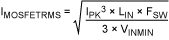(Eq. 11)

where IPK is the peak current calculated at the lowest operating input voltage, VINMIN.

MAX17498B/C has an internal MOSFET, the RMS current for the internal MOSFET can be calculated using the above formula.

### CCM Boost

In a CCM boost converter, the inductor current does not return to zero during a switching cycle. Since the MAX17597 and MAX17498B/C implement a nonsynchronous boost converter, the inductor current will enter DCM operation at load currents below a critical value, equal to half of the peak-to-peak ripple in the inductor current.

Inductor Selection

The design procedure for CCM boost starts with calculating the boost converter's input inductor at minimum input voltage. The inductor ripple current (LIR) can be chosen between 30% and 60% of the maximum input current.

 LIN = (VINMIN × DMAX × (1 - DMAX))/(LIR × IOUT × FSW) (Eq. 12)

where LIR is the chosen inductor ripple ratio (expressed in per unit) and DMAX, the duty cycle is calculated as:

 DMAX = (VOUT + VD - VINMIN)/(VOUT + VD) (Eq. 13)

VD is the voltage drop across the output diode of the boost converter at maximum output current.

Peak/RMS Current Calculation

For the purposes of setting current limit, the peak current in the inductor and MOSFET can be calculated as follows:

 IPK = [(VOUT × DMAX × (1 - DMAX))/(LIN × FSW) + (IOUT/(1 - DMAX))] for DMAX < 0.5 (Eq. 14)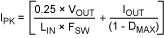for DMAX ≥ 0.5 (Eq. 15)

The value of current limit, in MOSFET N1, is set as:

 ILIM = IPK × 1.2 (Eq. 16)

In the case of the MAX17597, the current-sense resistor (RCS), connected between the source of the MOSFET N1 and PGND, sets the peak current limit. The current-limit comparator has a voltage trip level (VCS-PEAK) of 300mV. Use the following equation to calculate the maximum value of RCS:

 RCS = (300mV/ILIM)Ω (Eq. 17)

In the case of the MAX17498B/C the RLIM sets the current-limit value and its minimum value is:

 RLIM = ILIM × 50 in kΩ (Note that the worst-case ILIM is 1.62A for the MAX17498B/C)

Output Capacitor Selection

The output capacitance may be calculated as follows:

 COUT = 1/2 [(ISTEP × TRESPONSE)/ΔVOUT] (Eq. 18)

 TRESPONSE(0.33/FC) + (1/FSW) (Eq. 19)

where ISTEP is the load step, tRESPONSE is the response time of the controller, ΔVOUT is the allowable output voltage deviation, and fC is the target closed-loop crossover frequency. fC is chosen in the range of 1/10 to 1/5 RHP Zero.

 fRHP,Zero = (VOUT × (1 - DMAX)2)/(IOUT × 2 × π × LIN)

For a boost converter, the output capacitor supplies the load current when the main switch is ON, and therefore the output voltage ripple is a function of duty cycle and load current. Use the following equation to calculate the output capacitor steady-state ripple voltage:

 ΔVCOUT = (IOUT × DMAX)/(COUT × FSW) (Eq. 20)

Input Capacitor Selection

The minimum required input ceramic capacitor (C2) can be calculated based on the ripple allowed on the input DC bus.

 CIN = [(LIR × IOUT)/(8 × ΔVIN × fSW × (1 - DMAX)] (Eq. 21)

Where ΔVIN is the ripple voltage allowed on input DC bus.

In practice, an electrolytic capacitor (C1 of Figure 1) is provided to decouple any source inductance formed by the input cables. The electrolytic capacitor, C1, may also be used as an energy storage element, which can supply power when input power fails.

Capacitor values change with temperature and applied voltage. Refer to capacitor data sheets to select capacitors that would guarantee the required CIN and COUT values across the operating range. Use the worst case derated value of capacitance, based on temperature range and applied voltage, for further calculations.

Error Amplifier Compensation Design

The loop compensation values for the error amplifier may now be calculated as (for R4, C5, and C6):

 R4 = (182 × VOUT² × COUT × (1 - DMIN) × RCS)/(IOUT × LIN) for MAX17597 (Eq. 22)

 R4 = (46 × VOUT² × COUT × (1 - DMIN))/(IOUT × LIN) for MAX17498B/C (Eq. 23)

where DMIN is the duty cycle at the highest operating input voltage, given by the following expression.

 DMIN = (VOUT + VD - VINMAX)/(VOUT + VD) (Eq. 24)

 C5 = (VOUT × COUT)/(2 × IOUT × R4) (Eq. 25)

 C6 = 1/(π × FSW × R4) (Eq. 26)

Slope Compensation Ramp

The slope required to stabilize the converter at duty cycles greater than 50% can be calculated as follows:

 Se = ((0.82 × (VOUT - VINMIN) × RCS)/LIN)V/µs (Eq. 27)

Rcs = 0.5Ω for MAX1498B/C

where LIN is in µH. Refer to the MAX17597 or MAX1498B/C data sheet to set the R1 value for the required slope Se.

Output Diodes Selection

The design procedure for output-diode selection is identical to that outlined in the DCM Boost section.

MOSFET RMS Current Calculation

The voltage stress on the MOSFET ideally equals the sum of the output voltage and the forward drop of the output diode. In practice, voltage overshoot and ringing occur due to action of circuit parasitic elements during the turn-off transition. The MOSFET voltage rating should be selected with the necessary margin to accommodate this extra voltage stress. A voltage rating of 1.3 × VOUT provides the necessary design margin in most cases. The RMS current in the MOSFET is useful in estimating the conduction loss, and is given as:

 IMOSFETRMS = (IOUT × √DMAX)/(1 - DMAX) (Eq. 28)

where DMAX is the duty cycle at the lowest operating input voltage, and IOUT is the maximum load current. MAX17498B/C has an internal MOSFET, the RMS current for the internal MOSFET can be calculated using the above formula.

Feedback Potential Divider (Common Method for DCM and CCM Designs)

R2 and R3 of Figure 1 form the output voltage feedback network. Choose R2 = 10kΩ. Based on R2, calculate R3 as:

 R3 = R2 × (VOUT/1.21 - 1)kΩ (Eq. 29)

Refer to the MAX17597 or MAX1498B/C data sheet to program the soft-start duration, EN/UVLO, and OVI potential divider and switching frequency.